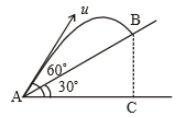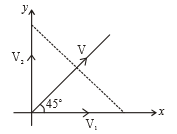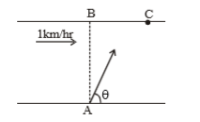A projectile is given an initial velocity of $\stackrel{^}{i}+2\stackrel{^}{j}$. The cartesian equation of its path is (g = 10 ${\mathrm{ms}}^{-2}$

1. $\mathrm{y}=2\mathrm{x}-5{\mathrm{x}}^{2}$

2. $\mathrm{y}=\mathrm{x}-5{\mathrm{x}}^{2}$

3. $4\mathrm{y}=2\mathrm{x}-5{\mathrm{x}}^{2}$

4. $\mathrm{y}=2\mathrm{x}-25{\mathrm{x}}^{2}$

Concept Questions :-

Projectile motion
High Yielding Test Series + Question Bank - NEET 2020

Difficulty Level:

A ship A is moving westwards with a speed of 10 km ${\mathrm{h}}^{-1}$ and a ship B, 100 km south of A is moving northwards with a speed of 10 km ${\mathrm{h}}^{-1}$. The time after which the distance between them becomes the shortest, is:

1.  5 hr

2.  $5\sqrt{2}$ hr

3.  $10\sqrt{2}$ hr

4.  0 hr

Concept Questions :-

Relative motion
High Yielding Test Series + Question Bank - NEET 2020

Difficulty Level:

Time taken by the projectile to reach from A to B is t. Then the distance AB is equal to :1. $\frac{ut}{\sqrt{3}}$

2. $\frac{\sqrt{3}ut}{2}$

3. $\sqrt{3}ut$

4. 2ut

Concept Questions :-

Projectile motion
High Yielding Test Series + Question Bank - NEET 2020

Difficulty Level:

A particle projected with kinetic energy ${\mathrm{k}}_{0}$ with an angle of projection $\mathrm{\theta }$. Then the variation of kinetic K with vertical displacement y is

1.  linear

2.  parabolic

3.  hyperbolic

4.  periodic

Concept Questions :-

Projectile motion
High Yielding Test Series + Question Bank - NEET 2020

Difficulty Level:

Three particles moving with constant velocities  and V respectively as given in the figure. After some time all three particles are in the same line, then relation among  and V is1.

2.

3.

4.

Concept Questions :-

Speed and velocity
High Yielding Test Series + Question Bank - NEET 2020

Difficulty Level:

A river is flowing with a speed of 1 km/hr. A swimmer wants to go to point 'C' starting from 'A'. He swims with a speed of 5 km/hr, at an angle $\theta$ w.r.t. the river. If AB=BC=400m. Then-(1) time taken by the man is 12 min

(2) time taken by the man is 8 min

(3) the value of $\theta$ is 45$°$

(4) the value of $\theta$ is 53$°$

Concept Questions :-

Relative motion
High Yielding Test Series + Question Bank - NEET 2020

Difficulty Level:

A body is thrown horizontally with a velocity $\sqrt{2gh}$ from the top of a tower of height h. It strikes the level ground through the foot of the tower at a distance x from the tower. The value of x is:

(1) h

(2) $\frac{h}{2}$

(3) 2h

(4) $\frac{2h}{3}$

Concept Questions :-

Projectile motion
High Yielding Test Series + Question Bank - NEET 2020

Difficulty Level:

A particle starts from the origin at t=0 and moves in the x-y plane with constant acceleration 'a' in the y direction. Its equation of motion is $y=b{x}^{2}$. The x component of its velocity (at t=0) is:

(1) variable

(2) $\sqrt{\frac{2a}{b}}$

(3) $\frac{a}{2b}$

(4) $\sqrt{\frac{a}{2b}}$

Concept Questions :-

Acceleration
High Yielding Test Series + Question Bank - NEET 2020

Difficulty Level:

A body projected with velocity u with an angle of projection $\theta$. Change in velocity after the time (t) from the projection is:

(1) gt

(2) $\frac{1}{2}g{t}^{2}$

(3) u sin$\theta$

(4) u cos$\theta$

Concept Questions :-

Projectile motion
High Yielding Test Series + Question Bank - NEET 2020

Difficulty Level:

A particle has initial velocity $\left(3\stackrel{^}{\mathrm{i}}+4\stackrel{^}{\mathrm{j}}\right)$ and has acceleration $\left(0.4\stackrel{^}{\mathrm{i}}+0.3\stackrel{^}{\mathrm{j}}\right)$. Its speed after 10 s

1. 7 units

2. $7\sqrt{2}$ units

3. 8.5 units

4. 10 units

Concept Questions :-

Uniformly accelerated motion